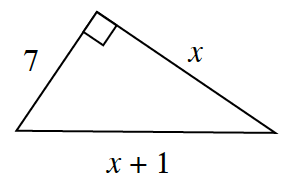### Home > CCG > Chapter 4 > Lesson 4.1.1 > Problem4-8

4-8.As Randi started to solve for $x$ in the diagram at right, she wrote the equation $7^2+x^2=(x+1)^2$ .

1. Is Randi’s equation valid? Explain your thinking.

Notice the right angle.

Yes, she used the Pythagorean Theorem.

2. To solve her equation, first rewrite $(x+1)^2$ by multiplying $(x+1)(x+1)$. You may want to review the Math Notes box for Lesson 2.2.2.

3. Now solve your equation for $x$.

Use a generic rectangle to multiply the binomials.

$x=24$

4. What is the perimeter of Randi’s triangle?

Use the value of $x$ to determine the length of each side of the triangle.